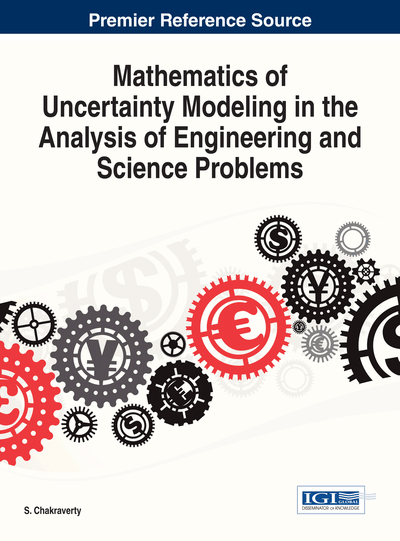# Numerical Solution of Fuzzy Differential Equations and its Applications

S. Chakraverty (National Institute of Technology - Rourkela, India) and Smita Tapaswini (National Institute of Technology – Rourkela, India)
DOI: 10.4018/978-1-4666-4991-0.ch007

## Abstract

Theory of fuzzy differential equations is the important new developments to model various science and engineering problems of uncertain nature because this theory represents a natural way to model dynamical systems under uncertainty. Since, it is too difficult to obtain the exact solution of fuzzy differential equations so one may need reliable and efficient numerical techniques for the solution of fuzzy differential equations. In this chapter we have presented various numerical techniques viz. Euler and improved Euler type methods and Homotopy Perturbation Method (HPM) to solve fuzzy differential equations. Also application problems such as fuzzy continuum reaction diffusion model to analyse the dynamical behaviour of the fire with fuzzy initial condition is investigated. To analyse the fire propagation, the complex fuzzy arithmetic and computation are used to solve hyperbolic reaction diffusion equation. This analysis finds the rate of burning number of trees in bounds where wave variable/ time are defined in terms of fuzzy. Obtained results are compared with the existing solution to show the efficiency of the applied methods.
Chapter Preview
Top

## 1. Introduction

Differential equations are extensively used in mathematical modelling and engineering applications. In order to make use of these, it is necessary to have solutions to the model equations. Generally, this requires numerical methods because of the complexity and number of equations. For the sake of simplicity the parameters and variables involved in the systems are considered as crisp or defined exactly. The solution of the differential equation with the variables and parameters as crisp can be handled either by exact methods or by known numerical methods developed by many authors. But we may have lack of information about the variables and parameters as those are obtained by some experiment or experience. Now-a-days these uncertainties are modelled through convex normalised fuzzy sets. So, we need to solve differential equations accordingly with fuzzy variables and parameters. Since, in real life applications, it is too complicated to obtain the exact solution of fuzzy differential equations, so the numerical methods are used to obtain the solution of fuzzy differential equations. As such, investigation of the fuzzy differential equations, in particular ordinary and partial differential equations by taking initial condition or boundary condition as fuzzy is an important area of research.

Chang and Zadeh (1972) first introduced the concept of a fuzzy derivative, followed by Dubois and Prade (1982), who defined and used the extension principle in their approach. The fuzzy differential equation and FIVP were studied by Kaleva (1987; 1990), Seikkala (1987) and others. Various numerical methods for solving fuzzy differential equations are given in [Ma et al. (1999); Friedman et al. (1999); Abbasbandy (2002); Abbasbandy et al.(2004); Mikaeilvand et al. (2012); Jayakumar, (2012); Tapaswini and Chakraverty (2012)]. Standard Euler method for solving fuzzy differential equations is introduced by Ma et al. (1999). A new numerical procedure investigated i.e. embedding method for solving FDEs and fuzzy integral equations (FIEs) with arbitrary kernels was developed by Friedman et al. (1999). Abbasbandy et al. (2002) applied taylor method for solving FDEs. Also Abbasbandy et al. (2004) developed a numerical method for solving linear and nonlinear fuzzy differential inclusions. A two-dimensional differential transform method to solve fuzzy partial differential equations (FPDEs) is proposed by Mikaeilvand et al. (2012). In this method fixed grid size is used to find approximate solutions of FPDEs. Also an adaptive grid size mechanism based on the fixed grid size technique is proposed. Based on the idea of improved Euler method Jayakumar (2012) investigated the numerical solution for hybrid fuzzy systems. Allahviranloo et al. [Allahviranloo et al. (2007; 2009)] introduced the predictor corrector method to FDEs. Javad Shokri (2007) applied modified Euler’s method for first order fuzzy differential equations and it’s an iterative solution. Duraisamy and Usha (2010) applied another approach to solve fuzzy differential equations by modified Euler’s method. Recently Tapaswini and Chakraverty (2012) have proposed a new method to solve fuzzy initial value problem. Bede and Gal (2005) studied general H-differentiability for fuzzy-number-valued function. Lia et al. (2012) developed the concept of generalized differentiability in a new way and applied the concept to solve for fuzzy differential equations. Buckley and Feuring (2001) applied two analytical methods for solving-th order linear differential equations with fuzzy initial conditions. Similarly many authors studied various other methods to solve-th order fuzzy differential equations in [Abbasbandy et al. (2011a; 2011b); Allahviranloo et al. (2008; 2009), Jafari(2012), Tapaswini and Chakraverty (2013)]. Malinowski (2009; 2012a, 2012b, 2012c) considered stochastic and random fuzzy differential equations to handle such type of uncertainties.

## Complete Chapter List

Search this Book:
Reset